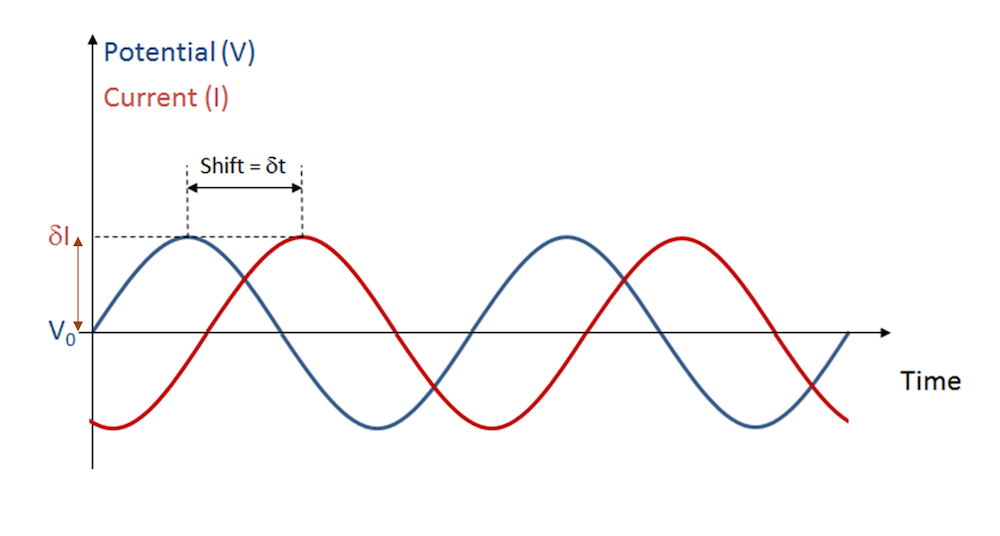# 电化学阻抗谱：实验、模型和仿真 App

2017年 2月 9日

### 电化学阻抗谱：实验V = V_0 \,\cos \left(\omega t\right)

I = I_0 \, \cos \left(\omega t + \phi \right)

R = \frac{V}{I} = \frac{V_0}{I_0} \,\frac{\cos(\omega t)}{\cos(\omega t + \phi)}

V = \mathrm{Re} \left{ V_0 \,\exp(i\omega t) \right\}

I = \mathrm\{Re} \left{ I_0 \,\exp(i\phi) \exp(i\omega t) \right\}

Z = \frac{\bar{V}}{\bar{I}} = \frac{V_0}{I_0} \,\exp(-i\phi)

EIS 专业人员以频谱的形式观察阻抗，通常使用奈奎斯特图，该图能够表现出阻抗虚部与实部的关系，在测量阻抗的每个频率下都有一个数据点。下图是一个仿真示例，我们将在下一节中讨论具体的建模过程。### 电化学阻抗谱：模型EIS 模型中 “电分析”接口的模型树。EIS 模型中“电极表面”边界特征的设置。